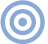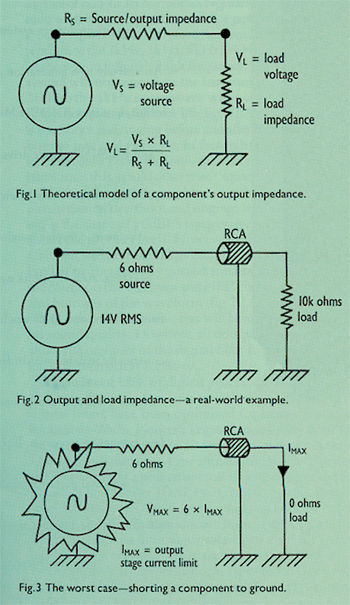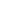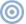# Features

## Quality Lies in the Details SIDEBAR 3: Output Impedance

SIDEBAR 3: Output Impedance
In the perfect world of mathematical abstraction, an electrical device such as a CD player or amplifier would deliver its signal in a manner completely unaffected by what it's hooked up to. In the real world, however, there's an interaction defined by the impedance of the load—the "load" or "input impedance"—and that of the source component—the "source" or "output impedance."

The output circuit, or stage, of a component can be mathematically modeled as a perfect, loss-less AC-voltage generator coupled to the outside world through a series resistor (fig.1). And it is that hypothetical series resistor that is equivalent to the output impedance. The signal has therefore to be shared between the load and source impedance. In a real-world example (fig.2), the Theta DS Pro Generation III has a measured output impedance of 6 ohms and a maximum output level of 14V RMS. This means that, into high impedances (100k ohms or above), the Theta will deliver pretty much the entire 14V. By Ohm's Law, the total current flowing is equal to 14V/(100,000+6), or 0.139992 milliamps (mA). Again by Ohm's Law, this current results in a voltage in the load impedance of 100,000 x 0.1399916 = 13,992mV = 13.9992V—a drop of just 0.8mV.

Into a much lower load impedance, however—a Jeff Rowland power amplifier, for example, which has an input impedance of 600 ohms—the Theta will deliver less than its maximum output voltage. The current flowing is now 14/(600+6) amps, or 23.1mA, meaning that the voltage available to the amplifier's input circuit is, by Ohm's Law, 600 x 23.1 = 13,861mV, or 13.861V.What if, instead of hooking up the Theta to a preamp, we short-circuit its output to ground (fig.3)? Now the full 14V will be developed across the Theta's 6 ohm output impedance, leading to more than 32W of power being dissipated if the output stage can deliver the necessary 2.33A of current. Of course it can't, but it's possible that, in trying to do so, the Theta's output devices will melt (footnote 1). Which is why it's unwise to short the output of a component being driven at its maximum level—unless you want to break it!—John Atkinson

Footnote 1: Actually, the BUF-03 chips used in the Theta DS Pro will protect themselves in the event of their outputs being short-circuited; but it's still unwise to do this unless no signal is being passed.—JAARTICLE CONTENTSX